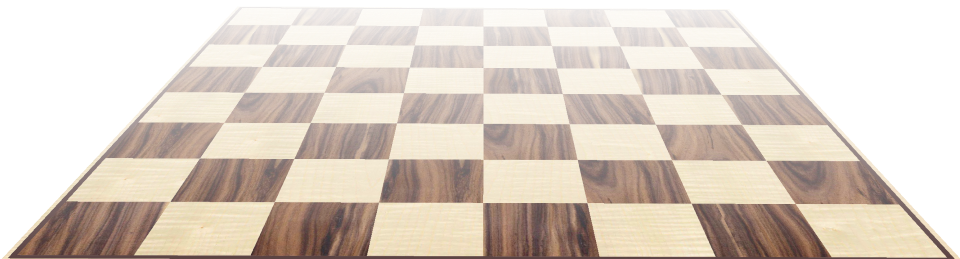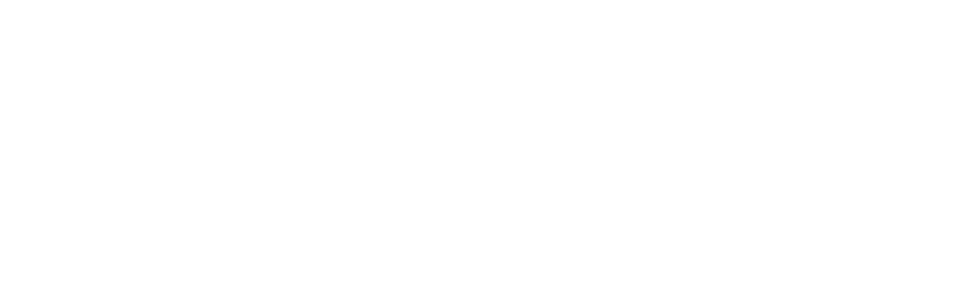# How many squares on a chess board?

How many squares are there on a chess board? Depending upon your interpretation, this can be perceived as a trick question. The answer of 64 squares (8×8), is perfectly valid, but there is also an alternative answer if we count the squares of different sizes, not just the individual squares.For instance, there is one square of size 8×8 units:Then there are four squares of size 7×7 units:And nine squares of size 6×6 units:I'm sure you've probably seen a pattern by now. As we progressively make the target square smaller by one unit, we can find this a perfect square number of times. There are 2×2 starting positions a (7×7) square can start from, there are 3×3 starting positions a (6×6) square can start from …The total number of squares is thus:There's also a formula for quickly calculating the sum of squares. For a chess board, with n=8, we can see the sum is 204.
Interestingly, as n gets larger, this formula tends to n3/3. I'm sure there is some particularly cool consequence of this, but I can't think of one at the moment. If you think of one, drop me a note, and I'll update this page and give you credit!

## Update!Image: Isabel encourages peace for all history students
Credit:Rachel

I received an nice email from Manos Kamarianakis who suggested that:

"A consequence of the sum of n2 tending to n3/3. It turns out to be quite simple and fascinating! Consider each number n2 to correspond to n2 little cubes of size 1, put in such way to form a square. For each n=1,2,3,… let us put this squares on top of each other (no need to centre them, thanks to Fubini's theorem). If we repeat this process infinitely, we can actually 'see' a pyramid forming, whose volume is indeed n3/3 = the sum of the cubes volume!"

I found a picture of such a Ziggurat, on the internet, made from sugar cubes!

## Rectangles

How about the number of rectangles on a chess board? Well, to make a rectangle you need to pick any two of the vertical lines, and any two of the horizontal lines. There are 9C2 pairs of horizontal lines you can pick (36 distinct pairs), and the same number of vertical pairs, so the answer is 36×36=1,296.
Here's a table of the the first few square grids, and the number of squares and rectangles:
Board Size# Squares# Rectangles
1×111
2×259
3×31436
4×430100
5×555225
6×691441
7×7140784
8×82041,296
9×92852,025
10×103853,025